## How to Construct an Equilateral Triangle?: Geometry

Happy Wednesday math peeps! This post introduces constructions by showing us how to construct an equilateral triangle by using a compass and a ruler. For anyone new to constructions, this is the perfect topic for art aficionados since there is more drawing than there is actual math.

## What is an Equilateral Triangle?

Equilateral Triangle: A triangle with three equal sides.  Not an easy one to forget, the equilateral triangle is super easy to construct given the right tools (compass+ ruler). Take a look below:

Now, for our Example:

## What’s Happening in this GIF?

1. Using a compass, measure out the distance of line segment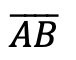.

2. With the compass on point A, draw an arc that has the same distance as.

3. With the compass on point B, draw an arc that has the same distance as.

4. Notice where the arcs intersect? Using a ruler, connect points A and B to the new point of intersection. This will create two new equal sides of our triangle!

Still got questions? No problem! Don’t hesitate to comment with any questions. Happy calculating! 🙂

Looking to learn more about triangles? Check out this post on right triangle trigonometry here!

## Expanding Cubed Binomials: Algebra 2/Trig.

Greetings math friends! This post will go over expanding cubed binomials using two different methods to get the same answer. We’re so used to seeing squared binomials such as,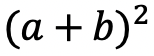, and expanding them without a second thought.  But what happens when our reliable squared binomials are now raised to the third power, such as,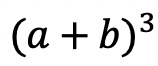?  Luckily for us, there is a Rule we can use:

But where did this rule come from?  And how can we so blindly trust it? In this post we will prove why the above rule works for expanding cubed binomials using 2 different methods:

Why bother? Proving this rule will allow us to expand and simplify any cubic binomial given to us in the future! And since we are proving it 2 different ways, you can choose the method that best works for you.

## Method #1: The Box Method

Step 1: First, focus on the left side of the equation by expanding (a+b)3:

Step 2: Now we are going to create our first box, multiplying (a+b)(a+b). Notice we put each term of (a+b) on either side of the box. Then multiplied each term where they meet.

Step 3: Combine like terms ab and ab, then add each term together to get a2+2ab+b2.

Step 4: Multiply (a2+2ab+b2)(a+b) making a bigger box to include each term.

Step 5: Now combine like terms (2a2b and a2b) and (2ab2 and ab2), then add each term together and get our answer: a3+3a2b+3ab2+b3.

## Method #2: The Distribution Method

Let’s expand the cubed binomial using the distribution method step by step below:

Now that we’ve gone over 2 different methods of cubic binomial expansion, try the following practice questions on your own using your favorite method!

Practice Questions: Expand and simplify the following.

Solutions:

Still, got questions?  No problem! Check out the video above or comment below! Happy calculating! 🙂

**Bonus: Test your skills with this Regents question on!

## How to use Recursive Formulas?: Algebra

Welcome to Mathsux! This post is going to show you everything you need to know about Recursive Formulas by looking at three different examples. Check out the video below for more of an explanation and test your skills with the practice questions at the bottom of this page.  Happy calculating! 🙂

## What is a Recursive Formula?

A Recursive Formula is a type of formula that forms a sequence based on the previous term value.  What does that mean?  Check out the example below for a clearer picture:

## Example #1:

-> First, let’s decode what these formulas are saying.

-> We found the sequence 2, 6, 10, 14, 18. Since we only needed the fifth term to answer our question, we know our solution is 18.

## Example #2:

-> First, let’s decode what these formulas are saying.

***Note this was written in a different notation but is solved in the exact same way!

-> We found the sequence 1,3,9. Since we only needed to find the third term to answer our questions, we know our solution is 9.

-> First, let’s decode what these formulas are saying.

-> We found the sequence 4,10, 28. Since we only needed to find the third term to answer our question, we know the solution is 28.

## Solutions:

Still got questions? No problem! Check out the video above for more or try the NYS Regents question below, and please don’t hesitate to comment with any questions. Happy calculating! 🙂

***Bonus! Want to test yourself with a similar NYS Regents question on Recursive Formulas?  Click here!

## Reflections: Geometry

Greetings and welcome to Mathsux! Today we are going to go over reflections, one of the many types of transformations that come up in geometry.  And thankfully, it is one of the easiest transformation types to master, especially if you’re more of a visual learner/artistic type person. So let’s get to it!

## What are Reflections?

Reflections on a coordinate plane are exactly what you think! When a point, a line segment, or a shape is reflected over a line it creates a mirror image.  Think the wings of a butterfly, a page being folded in half, or anywhere else where there is perfect symmetry.

## Example:

Step 1: First, let’s draw in line x=-2.

Step 2: Find the distance each point is from the line x=-2 and reflect it on the other side, measuring the same distance. First, let’s look at point C, notice it’s 1 unit away from the line x=-2, to reflect it we are going to count 1 unit to the left of the line x=-2 and label our new point, C|.

Step 3: Next we reflect point A in much the same way! Notice that point A is 2 units away on the left of line x=-2, we then measure 2 units to the right of our line and mark our new point, A|.

Step 4: Lastly, we reflect point B. This time, point B is 1 unit away on the right side of the line x=-2, we then measure 1 unit to the opposite side of our line and mark our new point, B|.

Step 5: Finally, we can now connect all of our new points, for our fully reflected triangle A|B|C|.

## Solutions:

Still got questions?  No problem! Check out the video above or comment below! Happy calculating! 🙂

Looking to review rotations about a point? Check out this post here!

## Piecewise Functions: Algebra

Greetings, today’s post is for those in need of a piecewise functions review!  This will cover how to graph each part of that oh so intimidating piecewise functions.  There’s x’s, there are commas, there are inequalities, oh my! We’ll figure out what’s going on here and graph each part of the piecewise-function one step at a time.  Then check yourself with the practice questions at the end of this post. Happy calculating! 🙂

## What are Piece-Wise Functions?

Exactly what they sound like! A function that has multiple pieces or parts of a function.  Notice our function below has different pieces/parts to it.  There are different lines within, each with their own domain.

Now let’s look again at how to solve our example, solving step by step:

Translation: We are going to graph the line f(x)=x+1 for the domain where x > 0

To make sure all our x-values are greater than or equal to zero, we create a table plugging in x-values greater than or equal to zero into the first part of our function, x+1.  Then plot the coordinate points x and y on our graph.

Translation: We are going to graph the line  f(x)=x-3 for the domain where x < 0.

To make sure all our x-values are less than zero, let’s create a table plugging in negative x-values values leading up to zero into the second part of our function, x-3.  Then plot the coordinate points x and y on our graph.

Ready to try the practice problems below on your own!?

## Practice Questions:

Graph each piecewise function:

## Solutions:

Still got questions?  No problem! Check out the video above or comment below for any questions. Happy calculating! 🙂

***Bonus! Want to test yourself with a similar NYS Regents question on piecewise functions?  Click here.

## Intersecting Secants Theorem: Geometry

Ahoy! Today we’re going to cover the Intersecting Secants Theorem!  If you forgot what a secant is in the first place, don’t worry because all it is a line that goes through a circle.  Not so scary right? I was never scared of lines that go through circles before, no reason to start now.

If you have any questions about anything here, don’t hesitate to comment below and check out my video for more of an explanation. Stay positive math peeps and happy calculating! 🙂

Wait, what are Secants?

Intersecting Secants Theorem: When secants intersect an amazing thing happens! Their line segments are in proportion, meaning we can use something called the Intersecting Secants Theorem to find missing line segments.  Check it out below:

Let’s now see how we can apply the intersecting Secants Theorem to find missing length.

Step 1: First, let’s write our formula for Intersecting Secants.

Step 2: Now fill in our formulas with the given values and simplify.

Step 3: All we have to do now is solve for x! I use the product.sum method here, but choose the factoring method that best works for you!

Step 4: Since we have to reject one of our answers, that leaves us with our one and only solution x=2.

Ready to try the practice problems below on your own!?

Practice Questions: Find the value of the missing line segments x.

Solutions:

Still got questions?  No problem! Check out the video above or comment below for any questions and follow for the latest MathSux posts. Happy calculating! 🙂

To review a similar NYS Regents question check out this post here.

## Absolute Value Equations: Algebra

Happy Wednesday math friends! Today, we’re going to go over how to solve absolute value equations.  Solving for absolute value equations supplies us with the magic of two potential answers since absolute value is measured by the distance from zero.  And if this sounds confusing, fear not, because everything is explained below!

Also, if you have any questions about anything here, don’t hesitate to comment. Happy calculating! 🙂

Absolute Value measures the “absolute value” or absolute distance from zero.  For example, the absolute value of 4 is 4 and the absolute value of -4 is also 4.  Take a look at the number line below for a clearer picture: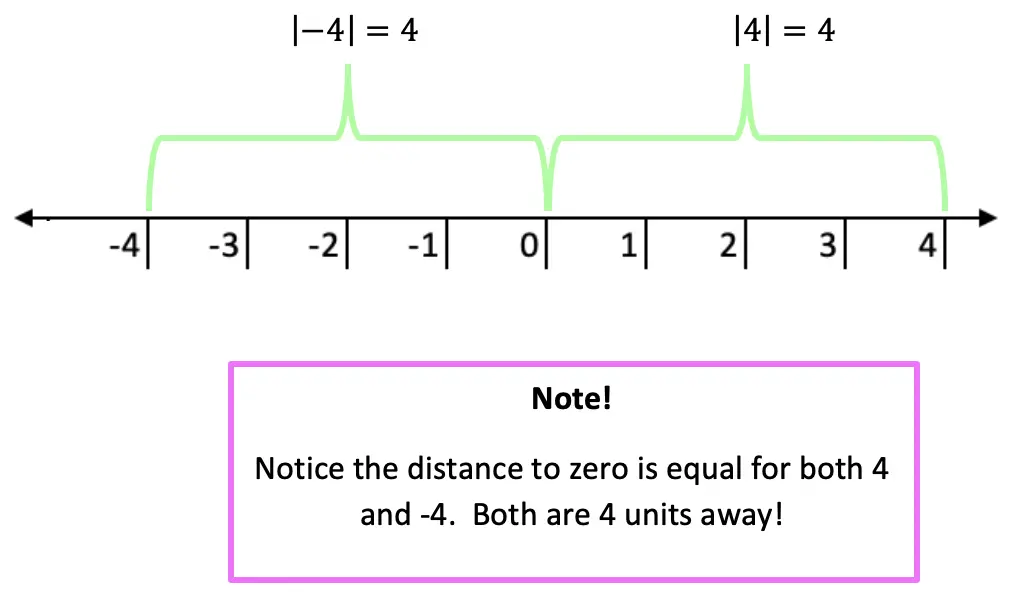Now let’s see how we can apply our knowledge of absolute value equations when there is a missing variable!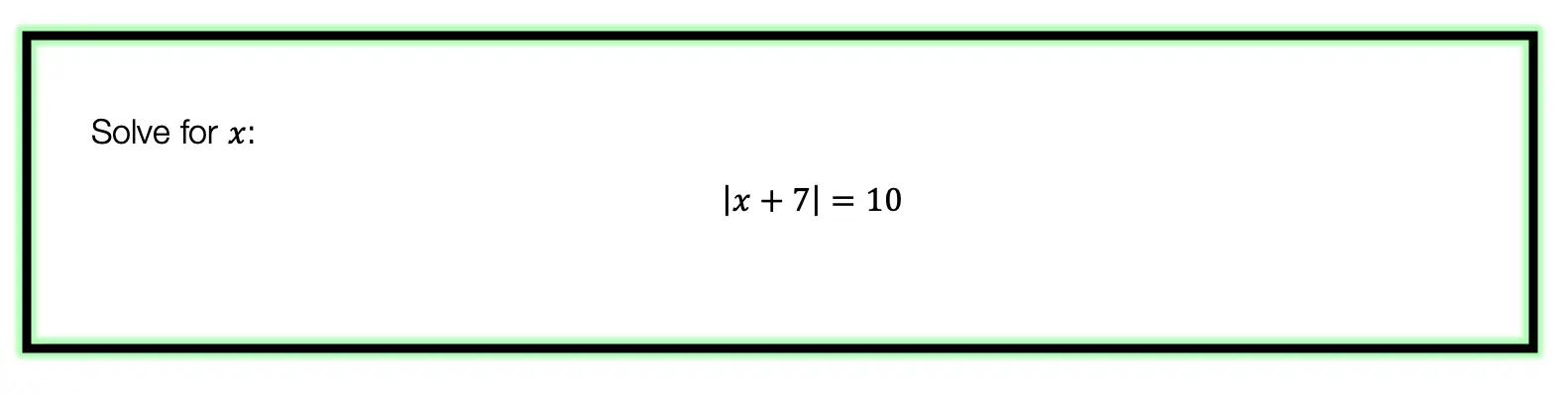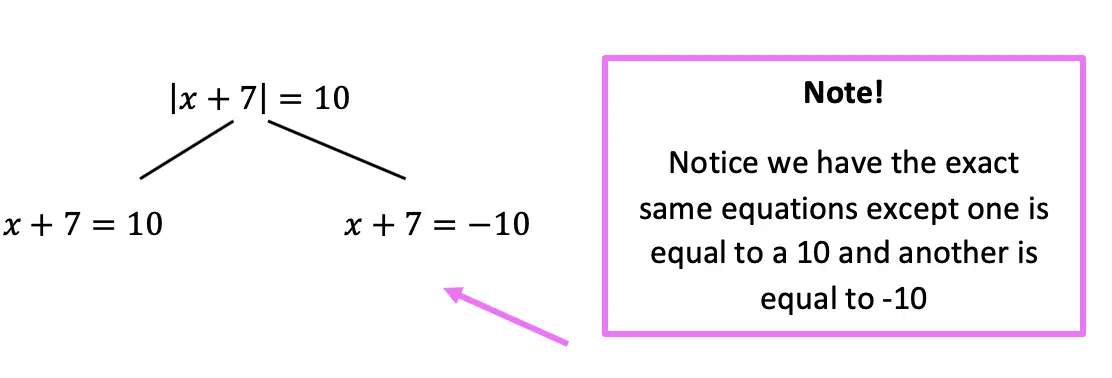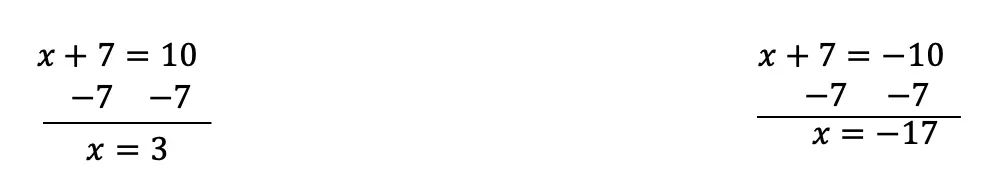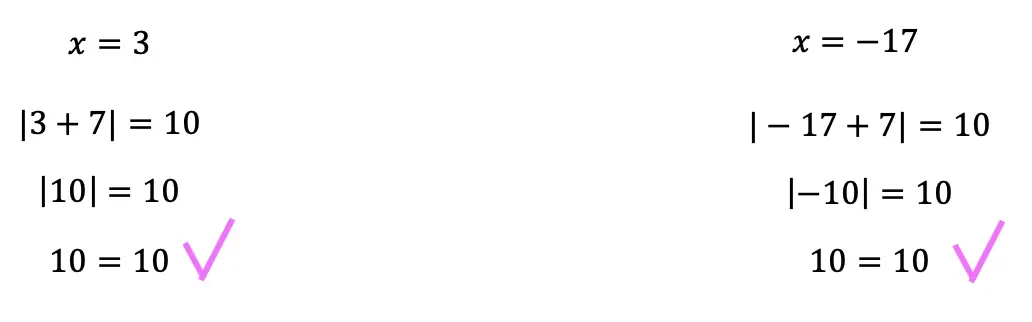Now let’s look at a slightly different example: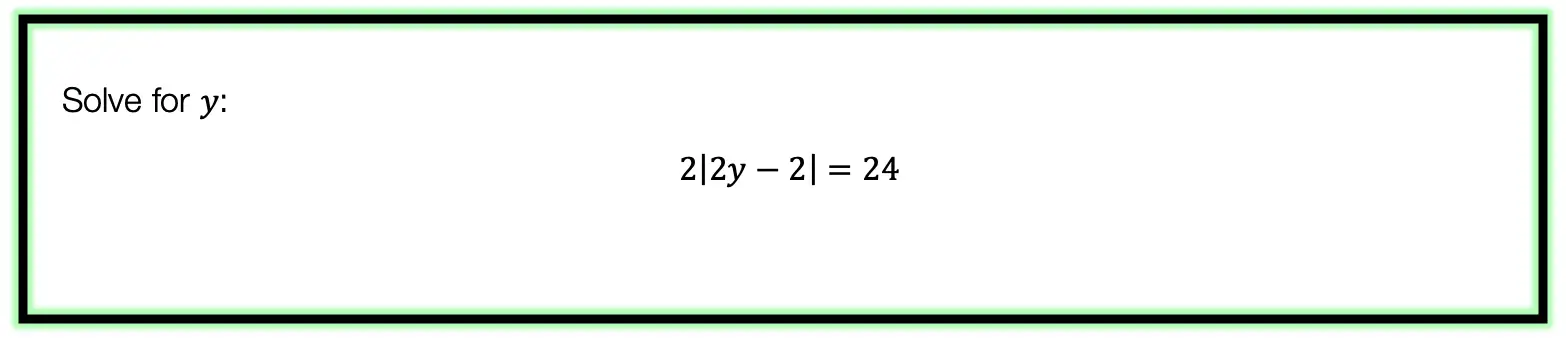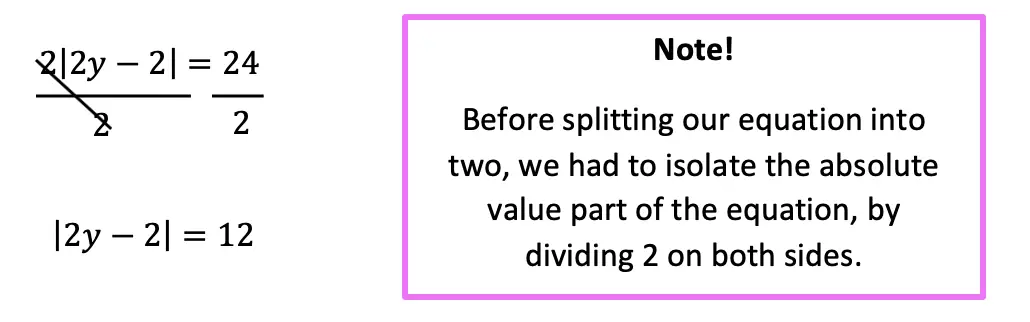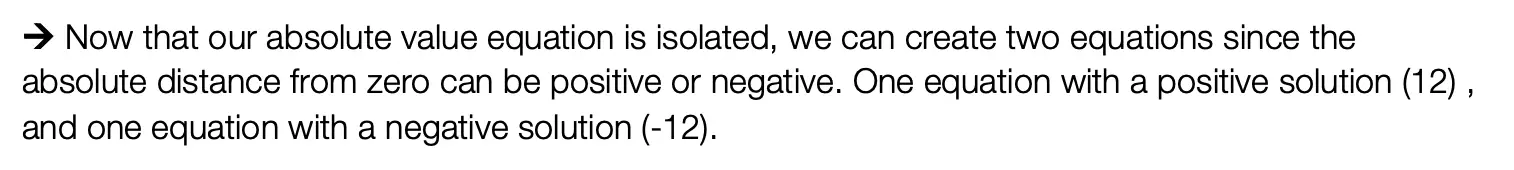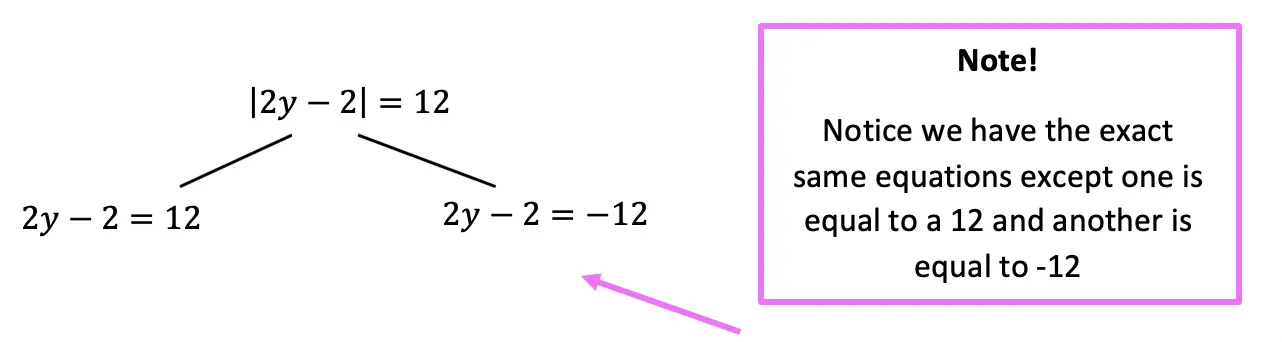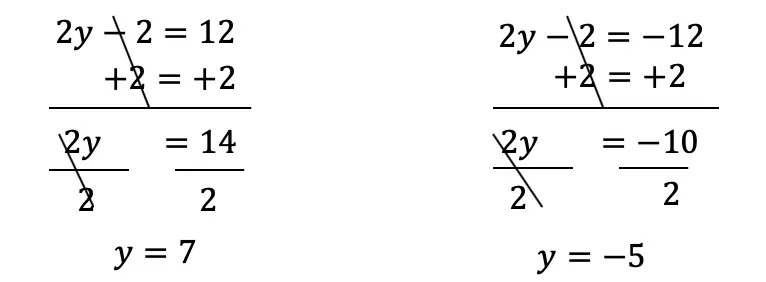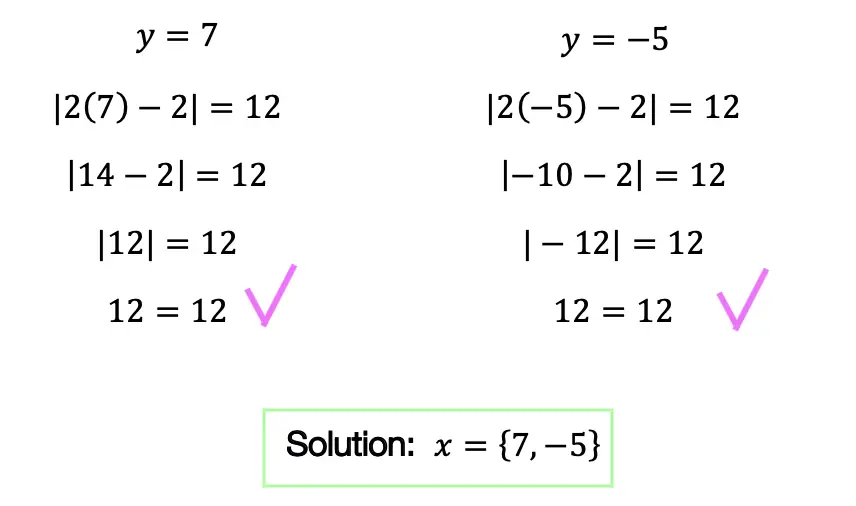Practice Questions: Given the following right triangles, find the missing lengths and side angles rounding to the nearest whole number.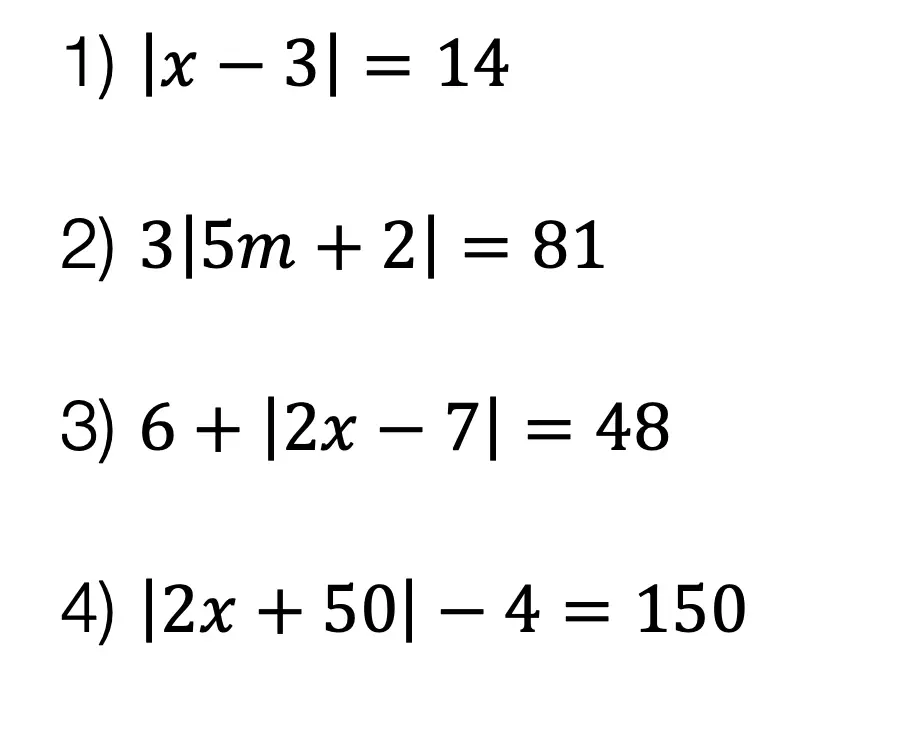Solutions: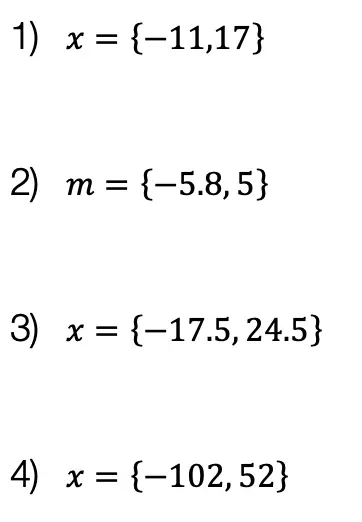Still got questions?  No problem! Check out the video the same examples outlined above. Happy calculating! 🙂

Also, if you’re looking for a review on combining like terms and the distributive property, check out this post here.

## How to use SOH CAH TOA: Geometry

Welcome back to Mathsux! This week, we’re going to go over how to find missing angles and side lengths of right triangles by using trigonometric ratios (sine, cosine, and tangent) (aka how to use SOH CAH TOA).  Woo hoo! These are the basics of right triangle trigonometry, and provides the base for mastering so many more interesting things in trigonometry! So, let’s get to it!

Also, if you have any questions about anything here, don’t hesitate to comment below or watch the video below. Also, don;r forget to subscribe to Math Sux for FREE math videos, lessons, and practice questions every week. Happy calculating! 🙂

## How to use SOH CAH TOA?

Trigonometric Ratios (more commonly known as Sine, Cosine, and Tangent) are ratios that naturally exist within a right triangle.  This means that the sides and angles of a right triangle are in proportion within itself.  It also means that if we are missing a side or an angle, based on what we’re given, we can probably find it!

Let’s take a look at what Sine, Cosine, and Tangent are all about!

Now let’s see how we can apply trig ratios when there is a missing side or angle in a right triangle!

Now for another type of question; using trig functions to find missing angles, let’s take a look:

Try the following Practice Questions on your own!

Solutions:

Still got questions?  No problem! Check out the video the same examples outlined above and happy calculating! 🙂

Need to brush up on special right triangles? Check out this posts on the 45 45 90 special triangles here!

## How to Solve Log Equations: Algebra 2/Trig.

Welcome to Mathsux! Today, we’re going to go over how to solve log equations, yay! But before we get into finding x, though, we need to go over what log equations are and why we use them in the first place…..just in case you were curious!

Also, if you have any questions about anything here, don’t hesitate to comment below or shoot me an email.  Happy calculating! 🙂

## What are Log Equations?

Logarithms are the inverses of exponential functions.  This means that when graphed, they are symmetrical along the line y=x.  Check it out below!

When on the same set of axis, notice how the functions are symmetrical over the line y=x:

We use logarithms to find the unknown values of exponents, such as the x value in the equation,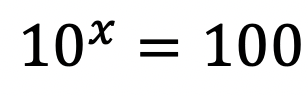.  This is a simple example, where we know the value of x is equal to 2,(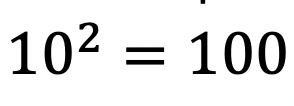). But what if it were to get more complicated?  That’s where logs come in!

## How to Solve Log Equations?

Logarithms follow a swooping pattern that allows us to write it in exponential form, let’s take a look at some Examples below:

But wait there’s more! Logs have a set of Rules that makes solving log equations a breeze!

We can use these rules to help us algebraically solve logarithmic equations, let’s look at an example that applies the Product Rule.

## Example:

Try the following practice questions on your own!

Practice Questions:

Solutions:

Still got questions?  No problem! Check out the video that goes over the same example outlined above.  And for more info. on logarithms check out this post that goes over a NYS Regent’s question here.  Subscribe below to get the latest FREE math videos, lessons, and practice questions from MathSux. Thanks for stopping by and happy calculating! 🙂

****Check out this Bonus Video on How to Change Log Bases****